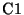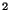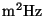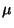Next: 14.21 Effective Solid Angles Up: 14. Calibration-G Products Previous: 14.19 PHT-S Wavelength and

Subsections

# 14.20 Conversion from Power on Detector to Flux Density


Description Parameter:	 Equations 5.10 and  5.11
Processing Level:	 AAR
Usage in processing:	 Section 8.4.6 and  8.4.7
File Names:		 PFLUXCONV


The transmission of the whole optical path for each filter is characterised by the parameterin mHz. The effective size of the primary mirror, reflections, filter transmission, spectral response of the detector, etc. are included. Thevalues for all filters are given in this calibration file. Also included are the reference wavelengths of the filter bands.

## 14.20.1 Product PFLUXCONV: PHT-P/C power calibration

PFLUXCONV is a FITS header without accompanying data. All entries listed here have unit:.


Keyword		Type	Description
FCP3P29		R	conversion for P1 3.3m filter
FCP3P6 		R	conversion for P1 3.6m filter
FCP4P85		R	conversion for P1 4.8m filter
FCP7P3 		R	conversion for P1 7.3m filter
FCP7P7 		R	conversion for P1 7.7m filter
FCP10 		R	conversion for P1 10m filter
FCP11P3		R	conversion for P1 11.3m filter
FCP11P5		R	conversion for P1 12m filter
FCP12P8		R	conversion for P1 12.8m filter
FCP16 		R	conversion for P1 15m filter
FCP20 		R	conversion for P2 20m filter
FCP25 		R	conversion for P2 25m filter
FCP60 		R	conversion for P3 60m filter
FCP100 		R	conversion for P3 100m filter
FCC50 		R	conversion for C100 65m filter
FCC60 		R	conversion for C100 60m filter
FCC70 		R	conversion for C100 80m filter
FCC90 		R	conversion for C100 90m filter
FCC100 		R	conversion for C100 100m filter
FCC105 		R	conversion for C100 105m filter
FCC120 		R	conversion for C200 120m filter
FCC135 		R	conversion for C200 150m filter
FCC160 		R	conversion for C200 170m filter
FCC180 		R	conversion for C200 180m filter
FCC200 		R	conversion for C200 200m filter


## 14.20.2 Product PFLUXCONV: PHT-P/C reference wavelengths

PFLUXCONV is a FITS header without accompanying data. All entries listed below have units: m.


Keyword		 Type	 Description
CWP3P29 	 R 	 reference wavelength of P1 3.3m filter (m)
CWP3P6 		 R 	 reference wavelength of P1 3.6m filter (m)
CWP4P85 	 R 	 reference wavelength of P1 4.8m filter (m)
CWP7P3 		 R 	 reference wavelength of P1 7.3m filter (m)
CWP7P7 		 R 	 reference wavelength of P1 7.7m filter (m)
CWP10 		 R 	 reference wavelength of P1  10m filter (m)
CWP11P3 	 R 	 reference wavelength of P1 11.3m filter (m)
CWP11P5 	 R 	 reference wavelength of P1 12m filter (m)
CWP12P8 	 R 	 reference wavelength of P1 12.8m filter (m)
CWP16 		 R 	 reference wavelength of P1  16m filter (m)
CWP20 		 R 	 reference wavelength of P2  20m filter (m)
CWP25 		 R 	 reference wavelength of P2  25m filter (m)
CWP60 		 R 	 reference wavelength of P3  60m filter (m)
CWP100 		 R 	 reference wavelength of P3 100m filter (m)
CWC50 		 R 	 reference wavelength of C100  65m filter (m)
CWC60 		 R 	 reference wavelength of C100  60m filter (m)
CWC70 		 R 	 reference wavelength of C100  80m filter (m)
CWC90 		 R 	 reference wavelength of C100  90m filter (m)
CWC100 		 R 	 reference wavelength of C100 100m filter (m)
CWC105 		 R 	 reference wavelength of C100 105m filter (m)
CWC120 		 R 	 reference wavelength of C200 120m filter (m)
CWC135 		 R 	 reference wavelength of C200 150m filter (m)
CWC160 		 R 	 reference wavelength of C200 170m filter (m)
CWC180 		 R 	 reference wavelength of C200 180m filter (m)
CWC200 		 R 	 reference wavelength of C200 200m filter (m)Next: 14.21 Effective Solid Angles Up: 14. Calibration-G Products Previous: 14.19 PHT-S Wavelength and
ISO Handbook Volume IV (PHT), Version 2.0.1, SAI/1999-069/Dc